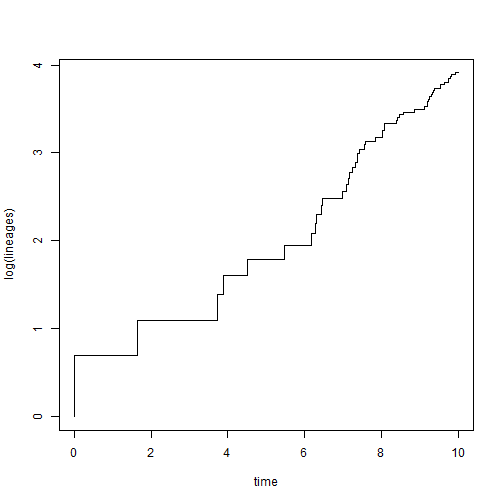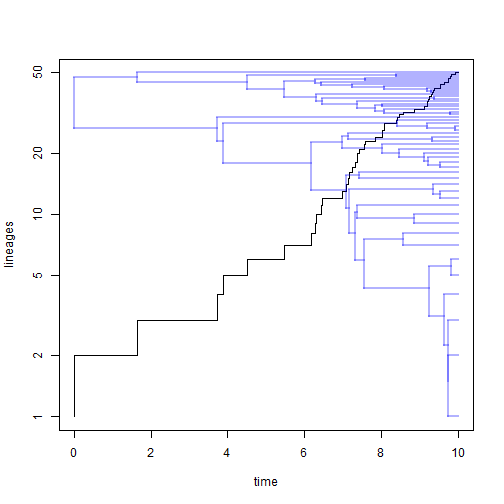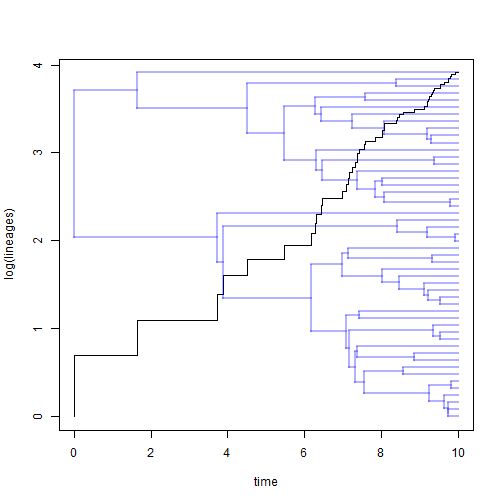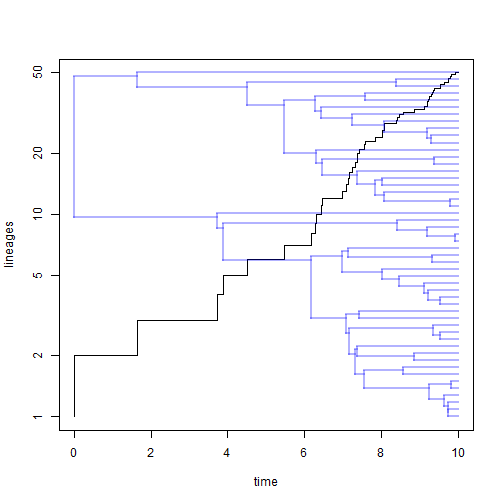## Monday, July 13, 2015

### Interesting feature of plot.ltt(...,log="y")Shortly after submitting the latest CRAN version of phytools, I discovered in interesting, unexpected “feature” of the new method to overlay a tree on a lineage-through-time plot using `ltt`.

The behavior appears when we set `log="y"` in the S3 plotting method for the object of class `"ltt"` in which we set `show.tree=TRUE`. If we do that, we see the following:

``````obj<-ltt(tree)
````````````plot(obj,log.lineages=FALSE,log="y",show.tree=TRUE)
``````At first, it looks OK - but then on closer inspection one quickly sees that the spacing of the edges is off. In fact, they have been plotted on a log, rather than a linear, scale. Oops! This occurs in spite of the fact that the lineage-through-time plot and the added phylogeny use different x & y scales in the standard plot as follows:

``````plot(obj,show.tree=TRUE)
``````I just posted an updated version of the code with this feature removed - however if users like it, I will post a workaround hack. I basically set the tip spacing of the plotted tree to `exp(1:Ntip(tree)` if `par()\$ylog==TRUE` as follows:

```if(show.tree){
tips<-if(par()\$ylog) setNames(exp(1:Ntip(x\$tree)),x\$tree\$tip.label)
else setNames(1:Ntip(x\$tree),x\$tree\$tip.label)
plotTree(x\$tree,color=rgb(0,0,1,transparency),
}
```

Let's try it:

``````source("http://www.phytools.org/ltt/v1.4/ltt.R")
plot(obj,show.tree=TRUE) ## still works
````````````plot(obj,log.lineages=FALSE,log="y",show.tree=TRUE) ## fixed
``````That's it.